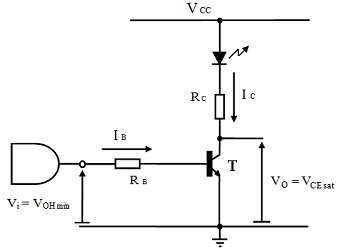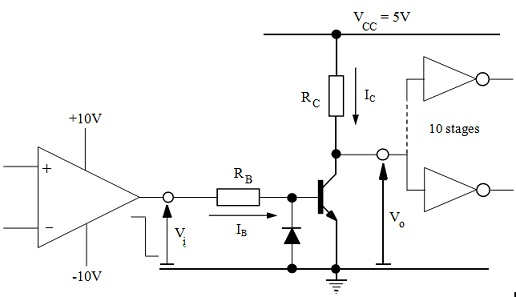#### Theory of Transistor Inverter Applications I

Introduction:

The simple transistor inverter circuit finds most of the applications, specifically in discrete form. It is much frequently employed as a current buffer where only a limited amount of current may be accessible, from the output of logic gate, for illustration, and more than this current is needed to drive some other element or component. This is too common in level shifting circuits to transform the logic levels from one system to other. It is generally used as a buffer to drive LEDs, relays, buzzers, solenoids and even small loudspeakers. A few of such applications will now be examined as worked illustrations.

A LED Buffer:

A buffer is needed to drive a red LED containing the properties given in graphs as shown in figures below. The brightness of 100mcd is needed from the LED whenever illuminated. The buffer is to be driven by the logic gate containing a VOH min = 0.8VCC operating from 5V supply. A bipolar transistor is accessible that has a minimum βF of 50, VBE sat = 0.8V and VCE sat = 0.1V.Figure: Schematic Diagram of LED BufferFigure: A Plot of the Luminous Intensity Properties of the LEDFigure: Electrical Characteristics of Light Emitting Diode

The schematic diagram of a proposed buffer is as shown in figure above. This differs from the single transistor inverter circuit only in that a LED is involved in the collector load and also the resistor, RC. The collector resistor serves to find out the level of current via the LED whenever the transistor is ON.

Step 1: Find out the forward current required in the LED to give the brightness level needed. Figure above shows the luminous intensity that can be interpreted as brightness, as a function of forward current in the diode. Coordinates of this curve for the 100mcd brightness are:

IV = 100mcd, IF = 30mA

Step 2: Find out the forward voltage drop across diode for the above value of current. Figure above exhibits the electrical current-voltage relationship from which it can be found for a current of 30mA. Coordinates are:

IF = 30mA, VD = 1.5V

Step 3: Compute the voltage drop across collector resistor, RC that is given as:

VRC = VCC – VD – VCE sat = 5V – 1.5V – 0.2V = 3.3V

Step 4
: The value of collector resistor can then be found as:

RC = VRC/IC = VRC/IF = 3.3/30 mA = 110 ?

Step 5: Check the power rating of resistor needed to make sure that it does not overheat. The power dissipated in RC is as follows:

PRC = VRC x IC = 3.3V x 30 mA = 100 mW

A resistor with 125mW rating could theoretically be employed. Though, it is a normal practice not to utilize circuit components at more than 75 to 80% of their power rating in order to decrease failure rates and extend their lifetime. Therefore, a resistor with power rating of 250mW would be employed to avoid being at this limit. It is the standard power rating for most of the resistors in low voltage circuits. The power rating of transistor is not critical as there is just a low voltage of VCE sat = 0.2V across it.

Step 6: The base current needed to drive the transistor must now be determined. This is primarily persuaded by βF of the transistor. To bring transistor to the edge of saturation whenever driving the LED needs a base current as:

IBEOS = ICF = IFF = 30 mA/50 = 0.6 mA

As the minimum value of βF has been employed, there is no requirement to permit for variations in this. Furthermore, the load conditions are fixed as simply a LED is to be driven. This signifies that really just temperature variations are present. This can be sufficiently permitted for by employing a base overdrive factor of 2. Then,

IB = σ IBEOS = σ (IFF) = 2 x (30 mA/50) = 1.2 mA

Step 7: Ultimately the value of base resistor RB, can be computed as:

RB = (VRB/IB) = (VOHmin – VBEsat)/IB = (0.8 x 5V – 0.8V)/1.2 mA = 3.2/1.2mA = 2.7 KΩ

A Level Shifting Circuit:

A circuit is needed to transform the HI and LO output levels of the comparator in threshold detector that operates from ±10V power supply to the logic levels of standard TTL family of gates operating from a single 5V supply. Each and every TTL gate input can be treated as containing an equivalent resistive load of 5kΩ joined between the supply rail and input. The level shifting circuit should provide a fan out of 10. The transistor is available that has a typical βF of 100 and a base-emitter reverse breakdown voltage of 5V.Figure: Schematic Diagram of the Level Shifting Circuit

Step 1: Find out the load current needed to drive 10 gates forming a load as outlined in specification. This can be done supposing a perfect logic LO output from the level shifting circuit. Then,

IL = (VCC - Vo)/(RL/N) = 5V/(5KΩ/10) = 10 mA

Step 2: Find out the total collector current required in the transistor. This can be completed by making an allowance for the current via a collector resistor RC beneath unloaded conditions. The value of RC of 10kΩ will permit a collector current of 0.5mA that is sufficient. This signifies that:

ICTOT = IC + IL = [(VCC - VO)/RC] + IL = 0.5 + 10 = 10.5 mA

Step 3: The base current can now be computed. To make sure that the transistor is at the edge of saturation needs a base current of:

ICF = 10.5 mA/100 = 105 μA

The value of βF employed is a typical value therefore this is subject to temperature variations and manufacturing process. The worst-case load conditions already have been accounted for in dealing with the fan-out needs and therefore a safety loaded overdrive factor of σL = 3 must suffice and hence:

IB = σL (ICF) = 3 x 105 μA = 315 μA

Step 4: Ultimately, the value of base resistor can be recognized. This is selected to permit the above value of base current to flow via it whenever being driven by a HI level input from comparator. Most of the comparators do not give an output voltage which goes to either supply rail. Those employing bipolar technology tend to provide an output voltage that is around 1 to 1.5V away from either supply rail voltage and this must be permitted for in computing the value of base resistor, RB. Then,

RB = (VOH min – VBE sat)/IB = [VCC (comp) – 1.5V – VBEsat]/IB = (10V – 1.5V – 0.8V)/315μA = 7.7V/315 μA = 24.4 KΩ

In this event, the closest value of 22kΩ in 5% manufacturing tolerance series would be employed.

Step 5: There is merely one remaining design detail which must be addressed. The specification states the base-emitter reverse breakdown voltage is of 5V. This signifies that the maximum reverse bias voltage that the base emitter junction can tolerate is of 5V (that is, max negative VBE = - 5V). Since the comparator is fed from bipolar ±10V supply, it’s LO output voltage will be of the order of - 8.5V that will reverse bias the base-emitter junction. As negligible current flows via the base resistor, RB, beneath this condition there is no voltage dropped across it and the whole 5V appears as a reverse bias across base emitter junction. This will surpass the maximum reverse bias permitted. The problem can be resolved by joining a diode across the base emitter junction in opposite direction to the junction itself as shown in figure above. Whenever the comparator output is HI this diode will itself be reverse biased (however will be selected to have a higher rating than transistor), the transistor turns ON and the level shifter output goes LO as needed. This can be inverted by first logic gate that is driven by the circuit. Whenever the comparator output goes LO, the transistor turns OFF and the level shifter output goes HI as needed. The diode conducts beneath this condition and limits the reverse bias across base-emitter junction to -VD = -0.5V.

There is, however, a comparator on the market that has the intrinsic ability to do level shifting. The LM311 marketed by the National Semiconductor Inc. gives an open-collector output phase. This is an output transistor which has no load joined to the collector that is simply brought to an output terminal on the chip and hence the user can join their own load. Similarly, the emitter of transistor is joined to a pin on the chip and hence users can select their own common or ground connection. This is as shown in figure below.Figure: The LM311

Latest technology based Electrical Engineering Online Tutoring Assistance

Tutors, at the www.tutorsglobe.com, take pledge to provide full satisfaction and assurance in Electrical Engineering help via online tutoring. Students are getting 100% satisfaction by online tutors across the globe. Here you can get homework help for Electrical Engineering, project ideas and tutorials. We provide email based Electrical Engineering help. You can join us to ask queries 24x7 with live, experienced and qualified online tutors specialized in Electrical Engineering. Through Online Tutoring, you would be able to complete your homework or assignments at your home. Tutors at the TutorsGlobe are committed to provide the best quality online tutoring assistance for Electrical Engineering Homework help and assignment help services. They use their experience, as they have solved thousands of the Electrical Engineering assignments, which may help you to solve your complex issues of Electrical Engineering. TutorsGlobe assure for the best quality compliance to your homework. Compromise with quality is not in our dictionary. If we feel that we are not able to provide the homework help as per the deadline or given instruction by the student, we refund the money of the student without any delay.